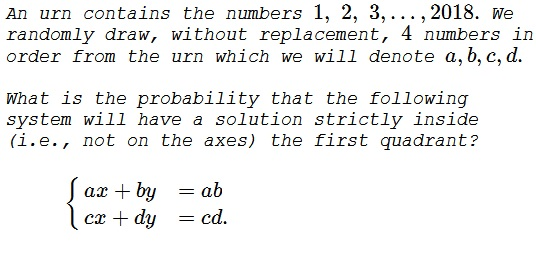# Probability of Random Lines Crossing

### Problem### Solution

The system is best rewritten as

$\displaystyle \begin{cases} \displaystyle \frac{x}{b}+\frac{y}{a}&=1\\\\ \displaystyle \frac{x}{d}+\frac{y}{c}&=1. \end{cases}$

In this form it is obvious that we deal with two straight lines, the first intersecting $x-$ and $y-\text{axes}$ at $(b,0)$ and $(0,a),$ respectively, whereas the second line crosses the axes at points $(d,0)$ and $(0,c),$ respectively. From a geometric view, the system never has a solution on the axes because the four numbers are distinct, although random. Thus the extra condition is a harmless red herring.

The two lines intersect within the first quadrant when $(a-c)(b-d)\lt 0.$ This condition is as probable as its complement $(a-c)(b-d)\gt 0.$ Thus both have the probability of $\displaystyle \frac{1}{2}.$

### Acknowledgment

This is problem 8 from the Wrangle contest at the Joint Mathematics Meetings 2018.Copyright © 1996-2018 Alexander Bogomolny

 64859831